Home » Mathematical programming – A basic example

# Mathematical programming – A basic example

Mathematical programming is a powerful tool to support decision making in a variety of fields including supply chain management and manufacturing. GAMS (General Algebraic Modeling System) is a software system that allows users to model and solve optimization problems using mathematical programming techniques. In this article, we will explore the basics of mathematical programming using GAMS in the context of a production example.

## The mathematical programming example

Suppose a company produces chairs and tables. Each chair requires 1 large board and 2 small boards, while each table requires 2 large boards and 2 small boards. The company has 5 large boards and 8 small boards available. The profit for selling a chair is 1000 dollars, and the profit for selling a table is 2500 dollars. The company wants to determine the optimal number of chairs and tables to produce in order to maximize profit while satisfying the available resources.

To solve this problem using GAMS, we need to define the decision variables, the objective function, and the constraints. Let’s use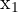to represent the number of chairs produced and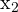to represent the number of tables produced. The objective function is to maximize the total profit, which can be expressed as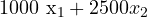. The constraints are the available resources, which can be expressed as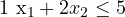(for the large boards) and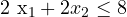(for the small boards).

We can represent this problem in GAMS as follows:

SETS
products /chairs, tables/;

PARAMETERS
profit(products) /chairs 1000, tables 2500/
available_large_boards /5/
available_small_boards /8/;

INTEGER VARIABLES
x(products);
VARIABLES
total_profit;

EQUATIONS
objective
large_boards
small_boards;

objective.. total_profit =E= sum(products, profit(products)*x(products));
large_boards.. 1*x('chairs') + 2*x('tables') =L= available_large_boards;
small_boards.. 2*x('chairs') + 2*x('tables') =L= available_small_boards;

MODEL m /all/;

SOLVE m USING MIP MAXIMIZING total_profit;

DISPLAY x.l, total_profit.l;

In this code, we first define a set of products that includes chairs and tables. We define parameters for the profit of each product and the available resources (large boards and small boards).

Next, we define the decision variables. We define an integer variable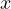as the production quantity of each product. We also define a variable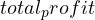for the profit of the manufacturer.

We define the equations of our model, which are the objective function and the constraints. The objective function calculated the total profit, which is the sum of the product of each product’s profit and the number of units produced. We also define two constraints, one for the available large boards and one for the available small boards.

We then define a model that includes all the variables, equations, and parameters we have defined. We set the optimization algorithm to MIP (mixed integer programming) and use the MAXIMIZING keyword to maximize the objective function.

We then use the DISPLAY statement to display the values ofandin the optimal solution.

## Output of GAMS

When we solve this model in GAMS, we get the following output:

----     29 VARIABLE x.L

chairs 1.000,    tables 2.000

----     29 VARIABLE total_profit.L        =     6000.000 

This output tells us that that the optimal solution is to produce 1 chair and 2 tables. This means that the company should produce 1 chair and 2 tables (since we cannot produce a fractional number of tables) to maximize profit while satisfying the resource constraints. The total profit in the optimal solution is \$6000.

## Conclusion

This simple example demonstrates how GAMS can be used to solve optimization problems in manufacturing. We model the problem as a mathematical program by defining parameters, decision variables, an objective function, and constraints, and use GAMS to find the optimal solution.

In more complex production problems, there may be additional constraints, such as production capacity, labor constraints, or demand constraints. GAMS can handle all additional constraints which makes it a powerful tool for solving complex manufacturing problems.